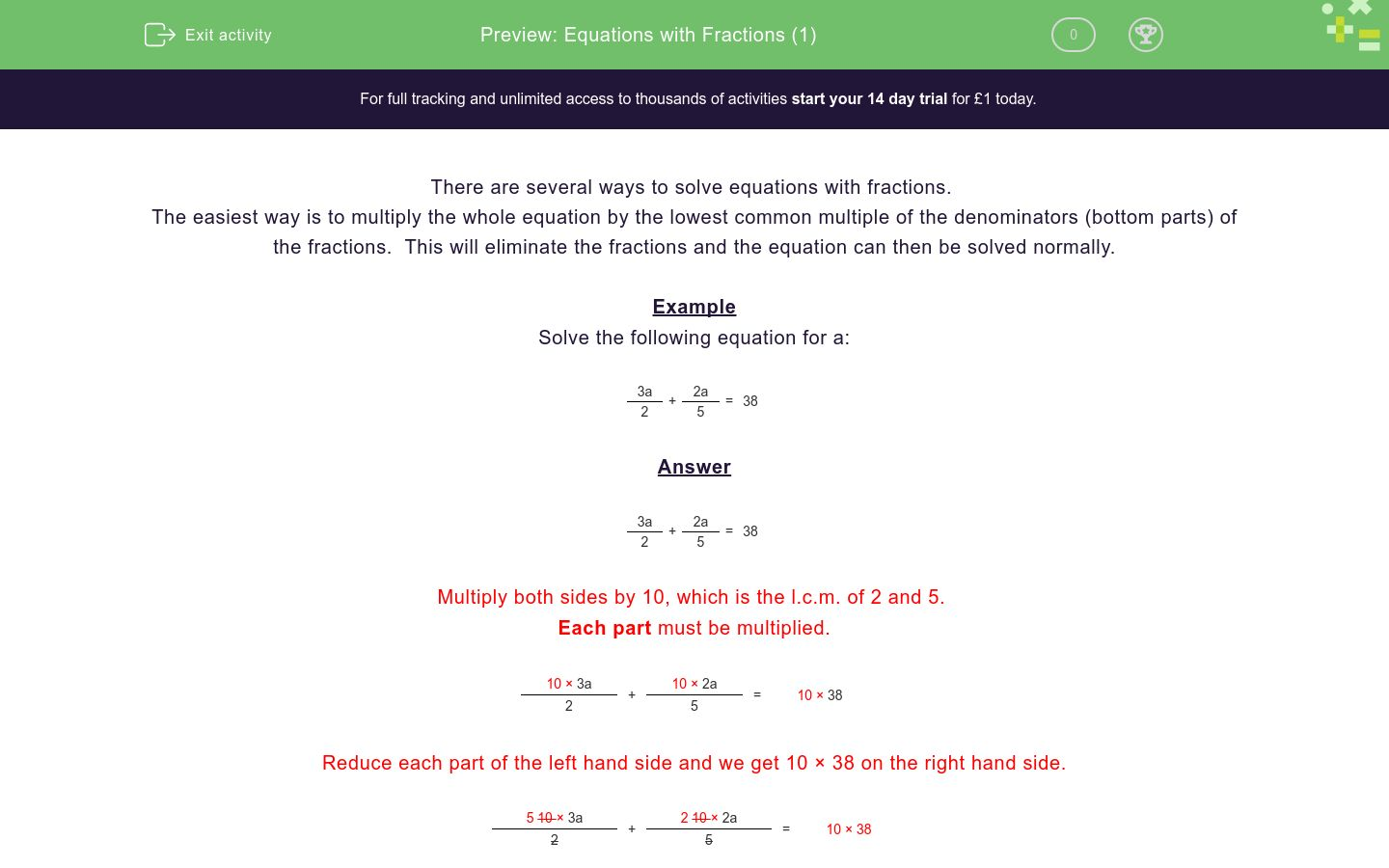# Equations with Fractions (1)

In this worksheet, students solve equations with fractions.Key stage:  KS 4

Curriculum topic:  Algebra

Difficulty level:### QUESTION 1 of 10

There are several ways to solve equations with fractions.

The easiest way is to multiply the whole equation by the lowest common multiple of the denominators (bottom parts) of the fractions.  This will eliminate the fractions and the equation can then be solved normally.

Example

Solve the following equation for a:

 3a + 2a = 38 2 5

 3a + 2a = 38 2 5

Multiply both sides by 10, which is the l.c.m. of 2 and 5.

Each part must be multiplied.

 10 × 3a + 10 × 2a = 10 × 38 2 5

Reduce each part of the left hand side and we get 10 × 38 on the right hand side.

 5 10 × 3a + 2 10 × 2a = 10 × 38 2 5

Simplify

5 × 3a + 2 × 2a = 380

Simplify again

15a + 4a = 380

Simplify again

19a = 380

Divide both sides by 19

19a ÷ 19 = 380 ÷ 19

Simplify

a = 20

Solve the following equation for a:

 3a + 2a = 12 2 4

(just write the value of a)

Solve the following equation for a:

 a + 2a = 18 2 5

(just write the value of a)

Solve the following equation for a:

 5a - 2a = 42 2 5

(just write the value of a)

Solve the following equation for a:

 a - 4a = 39 2 5

(just write the value of a)

Solve the following equation for a:

 3a + a = 23 2 7

(just write the value of a)

Solve the following equation for a:

 a - 5a = 3 2 9

(just write the value of a)

Solve the following equation for a:

 7a - 2a = 146 2 11

(just write the value of a)

Solve the following equation for a:

 2a + 3a = 93 3 11

(just write the value of a)

Solve the following equation for a:

 3a + 4a = 34 10 15

(just write the value of a)

Solve the following equation for a:

 2a - 11a = 14 3 12

(just write the value of a)

• Question 1

Solve the following equation for a:

 3a + 2a = 12 2 4

(just write the value of a)

6
EDDIE SAYS

l.c.m. is 4.

 4 x 3a + 4 x 2a = 4 x 12 2 4

Then simplify

2 x 3a + 2a = 48

• Question 2

Solve the following equation for a:

 a + 2a = 18 2 5

(just write the value of a)

20
EDDIE SAYS

l.c.m. is 10.

 10 x a + 10 x 2a = 10 x 18 2 5

Then simplify

5 x a + 2 x 2a = 180

• Question 3

Solve the following equation for a:

 5a - 2a = 42 2 5

(just write the value of a)

20
EDDIE SAYS

l.c.m. is 10.

 10 x 5a - 10 x 2a = 10 x 42 2 5

Then simplify

5 x 5a - 2 x 2a = 420

• Question 4

Solve the following equation for a:

 a - 4a = 39 2 5

(just write the value of a)

-130
EDDIE SAYS

l.c.m. is 10.

 10 x a - 10 x 4a = 10 x 39 2 5

Then simplify

5 x a - 2 x 4a = 390

• Question 5

Solve the following equation for a:

 3a + a = 23 2 7

(just write the value of a)

14
EDDIE SAYS

l.c.m. is 14.

 14 x 3a + 14 x a = 14 x 23 2 7

Then simplify

7 x 3a + 2 x a = 322

• Question 6

Solve the following equation for a:

 a - 5a = 3 2 9

(just write the value of a)

-54
EDDIE SAYS

l.c.m. is 18.

 18 x a - 18 x 5a = 18 x 3 2 9

Then simplify

9 x a - 2 x 5a = 54

-a = 54

a = -54

• Question 7

Solve the following equation for a:

 7a - 2a = 146 2 11

(just write the value of a)

44
EDDIE SAYS

l.c.m. is 22.

 22 x 7a - 22 x 2a = 22 x 146 2 11

Then simplify

11 x 7a - 2 x 2a = 3212

73a = 3212

• Question 8

Solve the following equation for a:

 2a + 3a = 93 3 11

(just write the value of a)

99
EDDIE SAYS

l.c.m. is 33.

 33 x 2a + 33 x 3a = 33 x 93 3 11

Then simplify

11 x 2a + 3 x 3a = 3069

31a = 3069

• Question 9

Solve the following equation for a:

 3a + 4a = 34 10 15

(just write the value of a)

60
EDDIE SAYS

l.c.m. is 30.

 30 x 3a + 30 x 4a = 30 x 34 10 15

Then simplify

3 x 3a + 2 x 4a = 1020

17a = 1020

• Question 10

Solve the following equation for a:

 2a - 11a = 14 3 12

(just write the value of a)

-56
EDDIE SAYS

l.c.m. is 12.

 12 x 2a - 12 x 11a = 12 x 14 3 12

Then simplify

4 x 2a - 11a = 168

-3a = 168

---- OR ----

Sign up for a £1 trial so you can track and measure your child's progress on this activity.

### What is EdPlace?

We're your National Curriculum aligned online education content provider helping each child succeed in English, maths and science from year 1 to GCSE. With an EdPlace account you’ll be able to track and measure progress, helping each child achieve their best. We build confidence and attainment by personalising each child’s learning at a level that suits them.